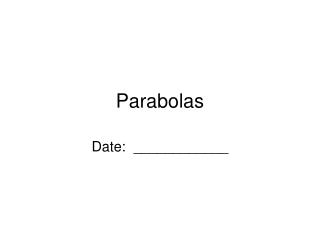DownloadDownload PresentationParabolas

# Parabolas

Télécharger la présentation## Parabolas

- - - - - - - - - - - - - - - - - - - - - - - - - - - E N D - - - - - - - - - - - - - - - - - - - - - - - - - - -
##### Presentation Transcript

1. Parabolas Date: ____________

2. Parabolas Parabola—Set of all points in a plane that are the same distance from a given point called the focus and a given line called the directrix. Focus Vertex Directrix

3. Parabolas with Vertex (0,0) that Open Up/Down 1 1 1 4a 4a 4a focus: (0,) directrix: y = – c = y = ax2 +a →opens up, -a → opens down vertex: (0, 0) c is the distance from the vertex

4. 1 1 1 4a 4a 4a focus: ( , 0) directrix: x = – c = Parabolas with Vertex (0,0) that Open Left/Right x = ay2 +a →opens right, -a → opens left vertex: (0, 0) c is the distance from the vertex

5. 1 1 4a 4a focus: (h, k + ) directrix: y = k – Parabola Opens Up/Down y – k = a(x – h)2 +a →opens up, -a → opens down vertex: (h, k)

6. Parabola Opens Left/Right 1 1 4a 4a focus: (h + , k) directrix: x = h – x – h = a(y – k)2 +a →opens right, -a → opens left vertex: (h, k)

7. 1 4a Write an equation of a parabola with a vertex at the origin and the given focus. x = ay2 opens to the right focus at (6,0) 4a · 6 = Solve for a · 4a 24a = 1 1 x = y² 24 24 24 1 a = 24

8. 1 4a Write an equation of a parabola with a vertex at the origin and the given directrix. x = ay2 opens to the left directrix x = 9 -4a · 9 = - Solve for a · -4a -36a = 1 1 x =- y² -36 -36 36 1 a = -36

9. 1 4a Write an equation of a parabola with a vertex at the origin and the given focus. y= ax2 opens up focus at (0,7) 4a · 7 = Solve for a · 4a 28a = 1 1 y = x² 28 28 28 1 a = 28

10. y x x = ⅛y2 Graph opens right vertex: (0, 0) 1 = 2 4(⅛) focus: (2,0) directrix: x = –2

11. Graph 1 12 y x y = - x2 opens down vertex: (0, 0) 1 = -3 1 4(- ) 12 focus: (0,-3) directrix: y = 3

12. y x y = ¼x2 Graph opens up vertex: (0, 0) 1 = 1 1 4( ) 4 focus: (0,1) directrix: y = -1

13. 1 16 y x x = - y2 Graph opens left vertex: (0, 0) 1 = -4 4(-1/16) focus: (-4,0) directrix: x = 4

14. Graph y – 3 = − (x + 3)2 1 16 y x opens down vertex: (-3, 3) 1 = -4 1 4(- ) 16 focus: (-3,-1) directrix: y = 7

15. Write the equation in standard form and graph the parabola.y2 – 8y + 8x + 8 = 0 y2 – 8y = -8x – 8 y2 – 8y + ___ = -8x – 8 16 +16 (y – 4)2 = -8x + 8 (y – 4)2 = -8(x – 1) -8 -⅛(y – 4)2 = x – 1 x – 1 = -⅛(y – 4)2

16. y x x – 1 = -⅛(y – 4)2 vertex: (1, 4) opens left 1 = -2 1 4(- ) 8 focus: (-1,4) directrix: x = 3

17. 1 6 1 6 Write the equation in standard form and graph the parabola.x2 – 6x + 6y + 18 = 0 x2 – 6x = -6y – 18 x2 – 6x + ___ = -6y − 18 +9 9 (x – 3)2 = -6y – 9 (x – 3)2 = -6(y + 1.5 ) -6 - (x – 3)2 = y +1.5 y + 1.5 = - (x − 3)2

18. y + 1.5 = - (x − 3)2 y x 1 6 vertex: (3,-1.5) opens down 1 = -1.5 1 4(- ) 6 focus: (3,-3) directrix: y = 0